# Automatically expand the VLOOKUP data rangeThe 2nd argument in the VLOOKUP function is the table_array.  This is the range of cells which contains the data to be used within the VLOOKUP function.    For example, in the code below A1:C9 is the table_array.

`=VLOOKUP("O Redding",A1:C9,3,FALSE)`

When using the VLOOKUP function, we often know how many rows there are in the data, therefore we know which cell references to include in the table_array.  But, there are also circumstances when additional rows will be added onto the existing data, therefore the exact data ranage is not known.  If you have experienced this, you probably followed one of the following methods:

• Included a table_array which is significantly bigger than required so that future data can be added (this method can result in slower calculation times).
• Updated the table_array argument each time the data changes to include the additional data (this method requires regular updates/changes to the VLOOKUP formula).
• Inserted the new rows into the existing table_array, rather than at the bottom (this method requires you to remember to insert them into the middle and not at the bottom.  Also, how will other users know to add the new data into the middle).

But, did you know there are other ways?  Ways, which once set-up, do not require the VLOOKUP function to be updated or the clever placement of the data.  In this post, we will consider these options:

1. Excel Tables
2. Dynamic named ranges

## Excel Tables

An Excel Table is an often misunderstood feature, but we won’t go into full detail here.  The key point for this post is that a Table expands or retracts automatically to fit the data.

### Table set-up

To set-up a Table, just highlight the cells and click Insert -> Table (Shortcut: Ctrl + T)The Create Table window will open.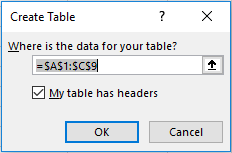Our data has headers and is within the range shown, so we can click OK.

The table has now formatted automatically to be striped (this can easily be changed if you so wish).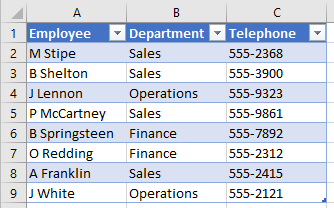We will rename the Table to a more useful name.  Click anywhere in the Table, then click Table Tools: Design in the Ribbon.  Rename the Table in the Table Name box.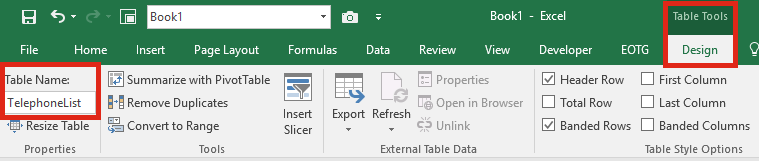### Using the Table

We can now use the “TelephoneList” Table as the table_array within the VLOOKUP.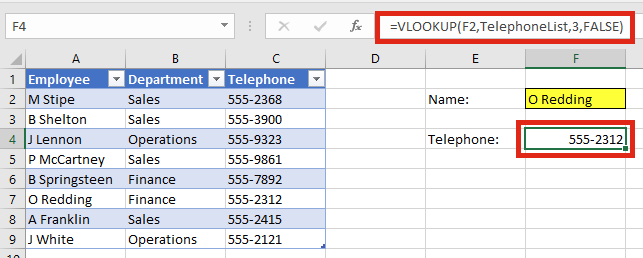Cell F4 includes the following formula:

`=VLOOKUP(F2,TelephoneList,3,FALSE)`

Each time new rows are added to the data, the source of the VLOOKUP will automatically be updated to include these cells.  In the screenshot below, B Adams has been added to Cells A10-C10 and “TelephoneList” has automatically updated too.  B Adams can now be found in the VLOOKUP withou needing to change the VLOOKUP formula.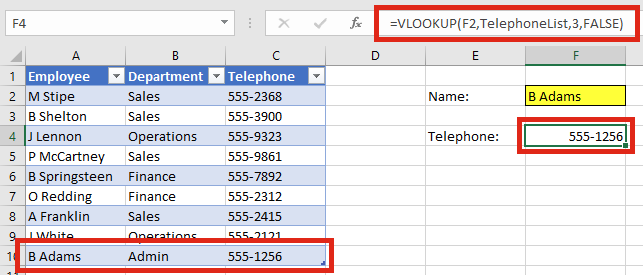## Dynamic named ranges

A named range is a group of cells which can be referred to by another name.  For example, you may click on Cells A1-C9 and give them the name “myRange”.   “myRange” can now be used within formulas instead of referencing A1-C9.

To set-up a named range you would use one of two methods

### Named range quick set-up

To quickly set-up a named range, just highlight the cells and type the name into the range box.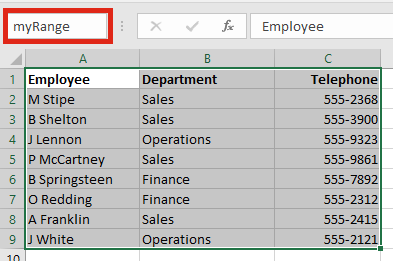### Defining the name

For a more detailed set-up, use the Define Name section of the Formulas Ribbon.

Using the menus click: Formulas -> Define Names -> Define Name.A new window will appear in which you can enter the information for your named range.  Then click OK.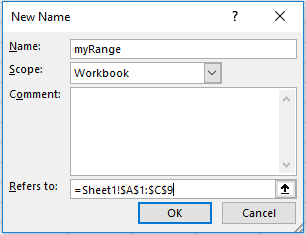### Setting up a dynamic named range

But we can go a step further.  We can use formulas to let Excel workout the size the named range should be, this is called a dynamic named range.  By using a dynamic named range, it will automatically expand to include any new data added to the bottom.

These dynamic named ranges experience issues when there are blank cells or where there is additional data inserted below the lookup table.  There are two different methods used below to tackle these two issues.

For either option setup the dynamic named range by defining the name.  But instead of using references to a range of cells, we will use the INDEX function to create the range.

OPTION 1: Base the range on the last used cell in a column.Look at function used in this example.

`=Sheet1!\$A\$1:INDEX(Sheet1!\$C:\$C,MAX((Sheet1!\$C:\$C<>"")*(ROW(Sheet1!\$C:\$C))))`

This is an Array Formula, so press Ctrl + Shift + Enter to enter the formula into the Refers to: box.

The formula starts with the sheet name and a reference to the first cell.  The INDEX function is used in conjunction with the MAX, and ROW functions to return the address of the last used cell in the column.  In the example above the INDEX function is returning a cell reference from Column C.

This formula still works where there are blank cells, but may create ranges bigger than necessary if there are any used cells below the lookup_data.

OPTION 2: Count the number of cells in a columnLook at function used in this example.

`=Sheet1!\$A\$1:INDEX(Sheet1!\$C:\$C,COUNTA(Sheet1!\$C:\$C))`

The formula starts with the sheet name and a reference to the first cell.  The INDEX function is then used in conjunction with the COUNTA functions to return the address based on the number of non-blank cells.  In the example above the INDEX function is returning a cell reference from Column C.

This formula does not work correctly where there are blank cells, as it may create a range smaller than necessary.

### Using a dynamic named range with VLOOKUP

We can now use “myRange” as the table_array within the VLOOKUP.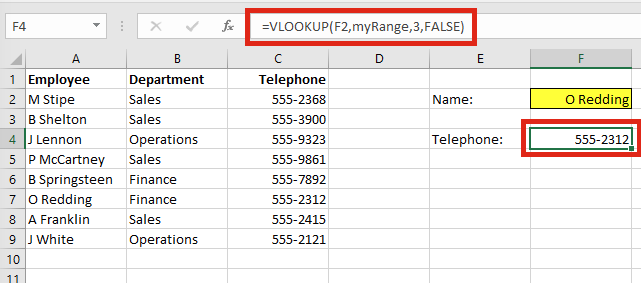Cell F4 includes the following formula:

`=VLOOKUP(F2,myRange,3,FALSE)`

Each time new rows are added to the data, the VLOOKUP will automatically be updated to include these cells.  B Adams has been added to Cells A10-C10 and “myRange” has automatically updated too.  B Adams can now be found in the VLOOKUP.You could keep adding data up to 500 rows and the VLOOKUP would work.  If you want more than 500 rows just update the cell references to the INDEX function within “myRange”.

Download the Advanced VLOOKUP Cheat Sheet.  It includes most of the tips and tricks we’ve covered in this series, including faster calculations, multiple criteria, left lookup and much more.

Please download it and pin it up at work, you can even forward it onto your friends and co-workers.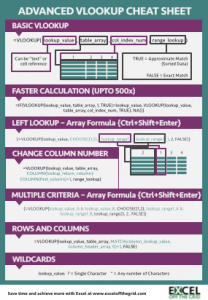Existing newsletter subscribers can enter their name and e-mail address into the form below for instant access to the download.  Or, you can also enter your name and e-mail address below to become a subscriber.

Save

## Want to Learn VBA & Macros?

If you want to automate Excel and save time, my recommended resouces for learning VBA Macros are:

### Books:

Excel 2016 Power Programming with VBA## 3 thoughts on “Automatically expand the VLOOKUP data range”

1.David says:

Interesting! I appreciate the Table explanation.

For the dynamic named range…you still have to adjust the reference if you wanted to go beyond 500, correct?

Why wouldn’t you just put the vlookup array to \$A\$1:\$C\$1000? If that were the case, what’s the benefit of the dynamic range?

•Excel Off The Grid says:

Hi David,

To be honest, I tend to use the Tables method rather than the dynamic named range method, it’s just much easier.

Dynamic named ranges of a completely unknown size have problems when used with blank cells or additional cells below the data table. I thought I had created a good solution, but you’re right, on second thoughts, there is little (if any) benefit from that approach. I have updated the article to show the two possible (but potentially flawed) dynamic named range approaches.

Thank you for taking the time to point this issue out to me.

2.JeteMc says:

Thank You!